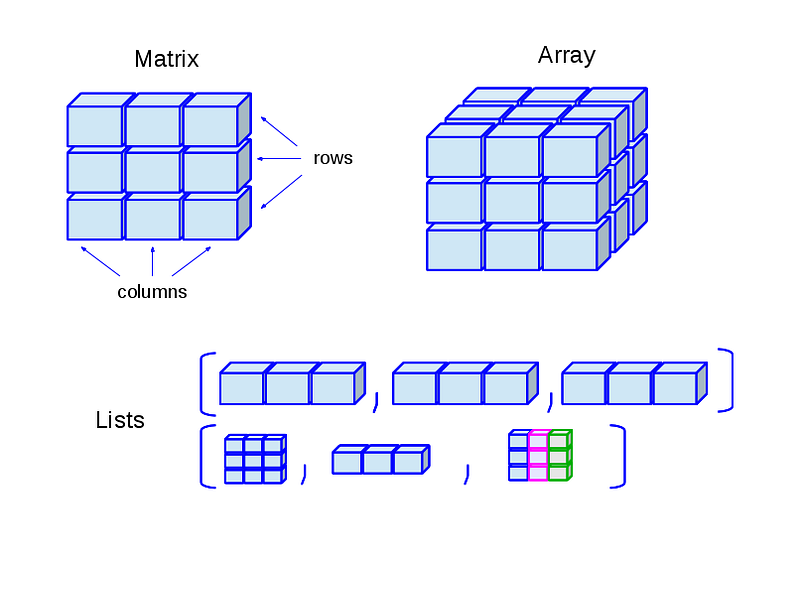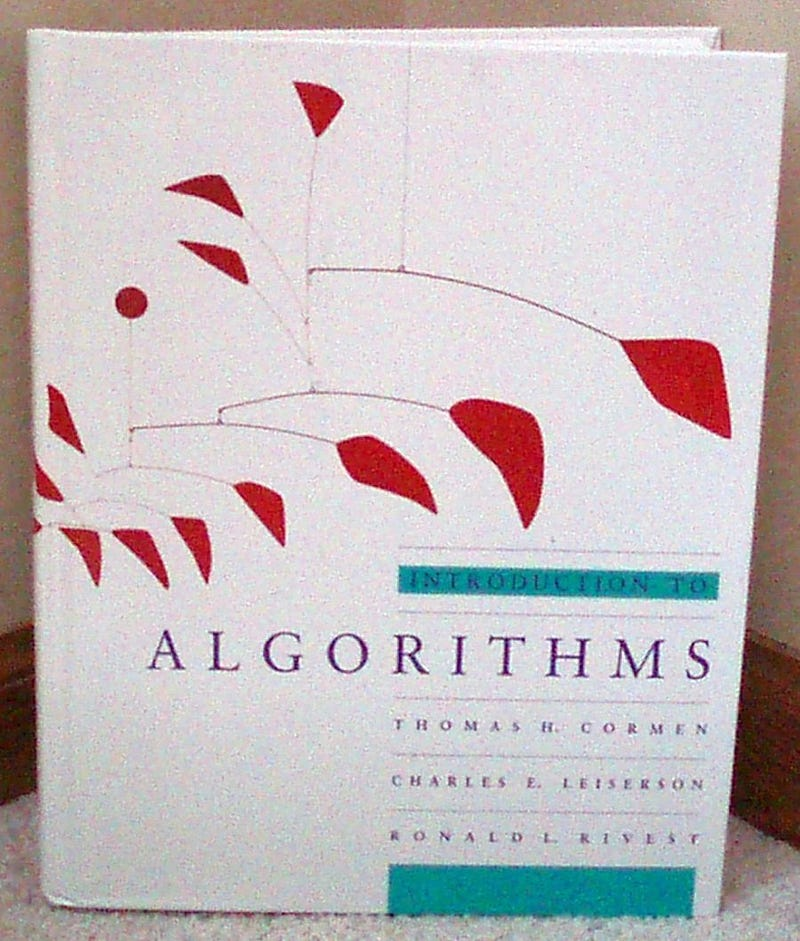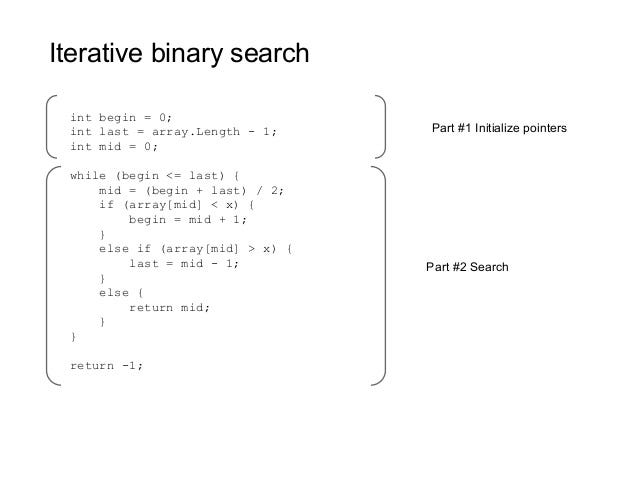# How to implement a Binary Search Algorithm in Java without recursion<br>

## An Iterative implementation of the popular binary search algorithm to find an element in a sorted array.

Hello everyone! I have published a lot of algorithms and data structure articles on my blog, but this one is the first one here. In this article, we’ll examine popular fundamental algorithms for interviews.

Yes, you guessed it right: you need to implement a binary search in Java, and you need to write both iterative and recursive binary search algorithms.

In computer science, a binary search, or half-interval search, is a divide and conquer algorithm that locates the position of an item in a sorted array. Binary searching works by comparing an input value to the middle element of the array.

The comparison determines whether the element equals the input, is less than the input, or is greater than the input.

When the element being compared equals the input, the search stops and typically returns the position of the element.

If the element is not equal to the input, then a comparison is made to determine whether the input is less than or greater than the element.

Depending on the result, the algorithm then starts over again, but only searching the top or a bottom subset of the array’s elements.

If the input is not located within the array, the algorithm will usually output a unique value indicating this like -1 or just throw a RuntimeException in Java like NoValueFoundException.

Binary search algorithms typically halve the number of items to check with each successive iteration, thus locating the given item (or determining its absence) in logarithmic time.

Btw, if you are not familiar with fundamental search and sort algorithms, then you can also join a course like Data Structures and Algorithms: Deep Dive Using Java to learn fundamental algorithms.If Java is not your choice of language, you can find more recommendations for JavaScript and Python in this list of algorithms courses.

Btw, if you prefer books, I suggest you read a comprehensive algorithm book like Introduction to Algorithms by Thomas H. Cormen.Here is some sample code which shows the logic of iterative binary search in Java:### Binary Search Implementation in Java

Here is a sample program to implement binary search in Java. The algorithm is implemented recursively. Also, an interesting fact to know about binary search implementation in Java is that Joshua Bloch, author of the famous Effective Java book, wrote the binary search in “java.util.Arrays”.

```import java.util.Arrays;
import java.util.Scanner;
/**
* Java program to implement Binary Search. We have implemented Iterative
* version of Binary Search Algorithm in Java
*
* @author Javin Paul
*/
public class IterativeBinarySearch {
public static void main(String args[]) {
int[] list = new int[]{23, 43, 31, 12};
int number = 12;
Arrays.sort(list);
System.out.printf("Binary Search %d in integer array %s %n", number, Arrays.toString(list));
binarySearch(list, 12);
System.out.printf("Binary Search %d in integer array %s %n", 43, Arrays.toString(list));
binarySearch(list, 43);
list = new int[]{123, 243, 331, 1298};
number = 331;
Arrays.sort(list);
System.out.printf("Binary Search %d in integer array %s %n", number,
Arrays.toString(list));
binarySearch(list, 331);
System.out.printf("Binary Search %d in integer array %s %n",   331, Arrays.toString(list));
binarySearch(list, 1333);
// Using Core Java API and Collection framework
// Precondition to the Arrays.binarySearch
Arrays.sort(list);
// Search an element
int index = Arrays.binarySearch(list, 3);
}
/**
* Perform a binary Search in Sorted Array in Java
* @param input
* @param number
* @return location of element in array
*/
public static void binarySearch(int[] input, int number) {
int first = 0;
int last = input.length - 1;
int middle = (first + last) / 2;
while (first <= last) {
if (input[middle] &lt; number) {
first = middle + 1;
} else if (input[middle] == number) {
System.out.printf(number + " found at location %d %n", middle);
break;
} else {
last = middle - 1;
}
middle = (first + last) / 2;
}
if (first > last) {
System.out.println(number + " is not present in the list.\n");
}
}
}
Output
Binary Search 12 in integer array [12, 23, 31, 43]
12 found at location 0
Binary Search 43 in integer array [12, 23, 31, 43]
43 found at location 3
Binary Search 331 in integer array [123, 243, 331, 1298]
331 found at location 2
Binary Search 331 in integer array [123, 243, 331, 1298]
1333 is not present in the list.
```

That’s all about how to implement binary search using recursion in Java. Along with Linear search, these are two of the essential search algorithms you learn in your computer science class.

The binary search tree data structure takes advantage of this algorithm and arranges data in a hierarchical structure so that you can search any node in O(logN) time.

Though, you must remember that in order to use binary search, you need a sorted list or array, so you also need to consider the cost of sorting when you consider using binary search algorithm in the real world.

Further Learning

10 Algorithms books for Interviews

10 Data Structure and Algorithm Courses for Interviews

5 Free Courses to Learn Data Structure and Algorithms

Other Data Structure and Algorithms tutorials you may like

• How to implement Quicksort algorithm in place in Java? (tutorial)
• How to implement Binary Search Tree in Java? (solution)
• How to implement Quicksort algorithm without recursion? (tutorial)
• How to implement Insertion sort algorithm in Java? (tutorial)
• How to implement Bubble sort algorithm in Java? (tutorial)
• What is the difference between Comparison and Non-Comparison based sorting algorithm? (answer)
• How to implement Bucket Sort in Java? (tutorial)
• How to implement a Binary Search Algorithm without recursion in Java? (tutorial)
• 50+ Data Structure and Algorithms Courses for Programmers (questions)

By :javinpaul

## Java Core (2020) | Java for beginners | Brush up your Java Skills | Get Hired

In this video we will learn about all the major concepts that come under Java Core . The course is very carefully designed and is made with keeping simplicit...

## Java Fundamentals: Learn Java for absolute beginners |Simpliv

Java Fundamentals: Learn Java for absolute beginners

## Searching Algorithms in Java

Searching Algorithms in Java. A Computer Science portal for geeks. It contains well written, well thought and well explained computer science and programming articles, quizzes and practice/competitive programming/company interview Questions.

## Data Structures and Algorithms - Advanced Java Programming Tutorial

Learn Data structure and algorithms in Java libraries. Java for Beginners : https://youtu.be/WqQsuIW2CFE C++ tutorial: https://youtu.be/mUQZ1qmKlLY Still Jav...

## Java Essentials : Learn Core Java From Basic to Advance

Learn Java Programming Using Practical Assignments. Start Building Back-end Web Applications Robust Test Automation Frameworks By End Of The Course. Learn More!